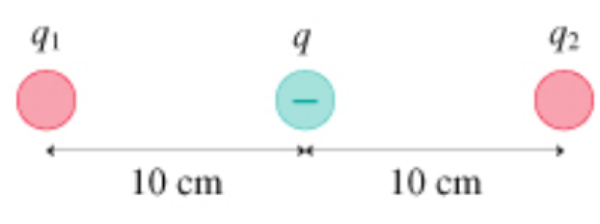# Problem: Charge q2 in the figure is in static equilibrium. Assume q = -8.1 nC.What is q1 in nC?

###### FREE Expert Solution

We're told that the charge q2 is in static equilibrium.

This means that the net force on q2 due to q and q1 must be equal.

Coulomb's law:

$\overline{){\mathbf{F}}{\mathbf{=}}\frac{\mathbf{k}{\mathbf{q}}_{\mathbf{1}}{\mathbf{q}}_{\mathbf{2}}}{{\mathbf{r}}^{\mathbf{2}}}}$, where k is Coulomb's constant and r is the distance between two charges q1 and q2.

The electrostatic force between q1 and q2:

99% (291 ratings)###### Problem Details

Charge q2 in the figure is in static equilibrium. Assume q = -8.1 nC.
What is q1 in nC?